# K-Means For Pair Selection In Python Part IIIBy Lamarcus Coleman

To Begin, we need to gather data for a group of stocks. We'll continue using the S&P 500. There are 505 stocks in the S&P 500. We will collect some data for each of these stocks and use this data as features for K-Means. We will then identify a pair within one of the clusters, test it for cointegration using the ADF test, and then build a Statistical Arbitrage trading strategy using the pair.

Let's get started!

### Part III: Building A StatArb Strategy Using K-Means

We'll begin by reading in some data from an Excel File containing the stocks and features will use.
```#Importing Our Stock Data From Excel
file=pd.ExcelFile('KMeansStocks.xlsx')#Parsing the Sheet from Our Excel file
stockData=file.parse('Example')```

Now that we have imported our Stock Data from Excel, let's take a look at it and see what features we will be using to build our K-Means based Statistical Arbitrage Strategy.

```#Looking at the head of our Stock Data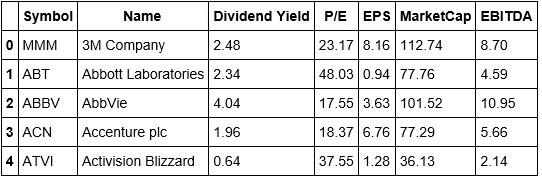```#Looking at the tail of our Stock Data
stockData.tail()```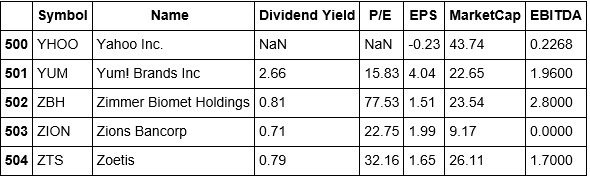We're going to use the Dividend Yield, P/E, EPS, Market Cap, and EBITDA as the features for creating clusters across the S&P 500. From looking at the tail of our data, we can see that Yahoo doesn't have a Dividend Yield, and is a missing P/E ratio. This brings up a good teaching moment. In the real world, data is not always clean and thus will require that you clean and prepare it so that it's fit to analyze and eventually use to build a strategy.

In actuality, the data imported as been preprocessed a bit as I've already dropped some unnecessary columns from it.

#### Process Of Implementing A Machine Learning Algorithm

Let's take a moment and think about the process of implementing a machine learning algorithm.
1. We begin by collecting our data
2. Next, we want to make sure our data is clean and ready to be utilized
3. In some cases, dependent upon what algorithm we're implementing, we conduct a train-test split( for K-Means this isn't necessary)
4. After conducting the train-test split, we then train our algorithm on our training data and then test it on our testing data
5. We then survey our model's precision

#### Implementation

Let's begin by cleaning up our data a little more. Let's change the index to the Symbols column so that we can associate the clusters with the respective symbols. Also, let's drop the Name column as it serves no purpose.

Before making additional changes to our data, let's make a copy of our original. This is a good practice as we could incur an error later and will be able to reset our implementation if we are working with a copy of the original instead of the original.

```#Making a copy of our stockdata
stockDataCopy=stockData.copy()#Dropping the Name column from our stockData
stockDataCopy.drop('Name', inplace=True,axis=1)```

It's a good practice to go back and check your data after making changes. Let's take a look at our stockData to confirm that we properly removed the Name column. Also, in the above line of code, we want to be sure that we include inplace=True. This states that the changes will persist in our data.

```#Checking the head of our stockData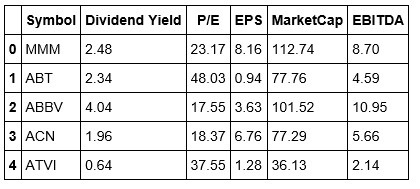Okay, now that we have properly dropped the Name column, we can change the index of our data to that of the Symbol column.

`stockDataCopy.reindex(index=stockDataCopy['Symbol'],columns=stockDataCopy.columns)`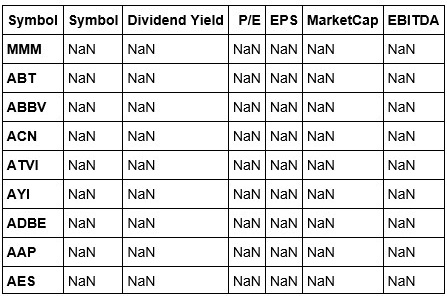We've reindexed our stockData, but this view isn't exactly what we were expecting. Let's fix this by adding the values back to our columns. We are able to do this because we are working using a copy of our original.
```#Adding back the values to our Columns
stockDataCopy['Symbol']=stockData['Symbol'].values
stockDataCopy['Dividend Yield']=stockData['Dividend Yield'].values
stockDataCopy['P/E']=stockData['P/E'].values
stockDataCopy['EPS']=stockData['EPS'].values
stockDataCopy['MarketCap']=stockData['MarketCap'].values
stockDataCopy['EBITDA']=stockData['EBITDA'].values```
We've added the data back to our stockDataCopy dataframe. Note in the code above, we were able to this because we could simply port over the values from our original dataframe. Let's take another look at our stock data.
```#Viewing the head of our stockDataCopy dataframeIt appears that Jupyter Notebook responds differently to reindexing and reassigning values to our dataframe that of the Spyder IDE. We won't worry about this for now but may need to create a workaround in the future. Now we will focus on clustering our data.

We begin by instantiating another K-Means object.

`stock_kmeans=KMeans()`

### Wait, How Do We Find K???

This brings us to another critical component of our strategy development. Recall, in our example of K-Means clustering, we created our own toy data and thus were able to determine how many clusters we would like. When testing the K-Means algorithm, we were able to specify K as 5 because we knew how many clusters it should attempt to create.

However, working with actual data, we are not aware of how many subgroups are actually present in our stock data. This means that we must identify a means of determining the appropriate amount of clusters, or value for K, to use. One such technique is to use termed the 'elbow' technique. We've mentioned this earlier, but I'll briefly recap. We plot the number of clusters versus the sum of squared errors, or SSE. Where the plot tends to bend, forming an elbow like shape, is the value of the clusters that we should select.

So, what we are tasked with doing, is to create a range of values for K, iterate over that range, and at each iteration fit our stock_kmeans model to our data. We will also need to store our K values and have a way to calculate the distances from the centroids of each iteration so that we can compute our SSE or sum of squared errors.

To find our distances, we'll use scipy. Let's import it now.

`from scipy.spatial.distance import cdist`

If you would like to learn more about the cdist object you can visit this link. The distance used in K-Means is the Euclidean distance and this is the one we will use with this method.

Let's create our elbow chart to determine the value of K.

```#creating an object to determine the value for K

class Get_K(object):
def __init__(self,start,stop,X):
self.start=start
self.stop=stop
self.X=X
#in our example, we found out that there were some NaN
#values in our data, thus we must fill those with 0
#before passing our features into our model
self.X=self.x.fillna(e)

def get_k(self):
#this method will iterate through different
#values of K and create the SSE
#initializing a list to hold our error terms
self.errors=[ ]
#intializing a range of values for K
Range=range(self.start,self.stop)
#iterating over range of values far K
#and calculating our errors
for i in Range:
self.k_means=KMeans(n_clusters=i)
self.k_means.fit(self.X)
self.errors.append(sum(np.min(cdist(self.X[0:200],self.k_means.cluster_centers_,'euclidean'),axis=1))/200)
return

def plot_elbow(self):
with plt.style.context(['seaborn-notebook','ggplot‘]):
plt.figure(figsize=(10,8))
#we have multiple features, thus we will use the
#P/E to create our elbow
plt.plot(self.X['P/E'][0:200],self.errors[0:200])
plt.xlabel('Clusters')
plt.ylabel('Errors')
plt.title('K-Means Elbow Plot')
plt.tight_layout()
plt.show()
return```

We now have an object to determine the value we should use for K. We will create an instance of this object and pass in our stockData and determine the value we should use for K.

Let's first create a list of our features.

`features=stockDataCopy[[‘Dividend Yield','P/E','EPS','MarketCap','EBITDA']]`

Now that we have set our features, we can pass them into our K-Means algorithm.

```#Creating an instance of our Get_K object#we are setting our range of K from 1 to 266
#note we pass in the first 200 features values in this example
#this was done because otherwise, to plot our elbow, we would
#have to set our range max at 500. To avoid the computational
#time associated with the for loop inside our method
#we pass in a slice of the first 200 features#this is also the reason we divide by 200 in our class

Find_K=Get_K(1, 200,features [1:200]```

At this point, we have created our list of features, and have created an instance of our Get_K class with a possible range of K from 1 to 200. Now we can call our get_k method to find our errors.

```#Calling get_k method on our Find_K object
Find_K.get_k()```

### Visualizing K-Means Elbow Plot

Now that we have used our get_k method to calculate our errors and range of K, we can call our plot_elbow method to visualize this relationship and then select the appropriate value for K.
```#Visualizing our K-Means Elbow Plot
Find_K.plot_elbow()```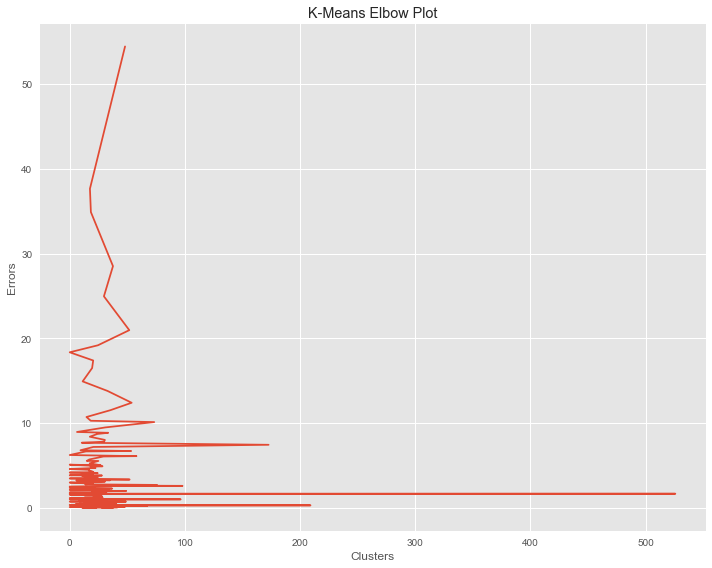We can now use the above plot to set our value for K. Once we have set K, we can apply our model to our stock data and then parse out our clusters and add them back to our stockData dataframe. From here we are then able to manipulate our dataframe so that we can identify which stocks are in which cluster. Afterwards, we can select pairs of stocks and complete our analysis by checking to see if they are cointegrated and if so build out a Statistical Arbitrage strategy.

In this post, we have continued to build on our understanding of how K-Means can improve our Statistical Arbitrage strategies. We learned that an important problem to solve with implementing K-Means is determining what value we should use for K. In the last post in this series we will use what we have learned up to this point to build an actual Statistical Arbitrage strategy based solely on pairs composed by our K-Means algorithm. Let’s finish up!

### Next Step

Learn about K-Means clustering, its advantages, and its implementation for Pair Selection in Python. Create a Statistical Arbitrage strategy using K-Means for pair selection and implementing the elbow technique to determine the value of K in our post 'K-Means Clustering For Pair Selection In Python – Part I'.

Disclaimer: All investments and trading in the stock market involve risk. Any decisions to place trades in the financial markets, including trading in stock or options or other financial instruments is a personal decision that should only be made after thorough research, including a personal risk and financial assessment and the engagement of professional assistance to the extent you believe necessary. The trading strategies or related information mentioned in this article is for informational purposes only.